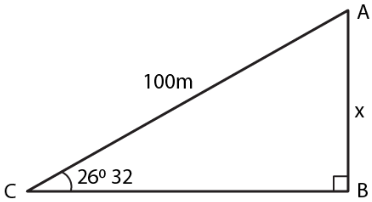Guru

# A boy is flying a kite with a string of length 100 m. If the string is tight and the angle of elevation of the kite is 260 32’, find the height of the kite correct to one decimal place, (ignore the height of the boy).

• 0

sir this is the question from the book -ML aggarwal( avichal publication) class 10th ,
chapter20 , heights and distances
we have been given as a information that a boy is flying a kite with a string of length 100 m.
If the string is tight and the angle of elevation of the kite is 260 32’,
now we have to find the height of the kite correct to one decimal place, (ignore the height of the boy).

question no 8 , heights and distances , ICSE board, ml aggarwal

Share

1. Consider AB as the height of the kite A and AC as the string

Angle of elevation of the kite = 260 32’Take AB = x m and AC = 100 m

We know that

sin θ = AB/AC

Substituting the values

sin 260 32’ = x/100

So we get

0.4467 = x/100

By further calculation

x = 100 × 0.4467

x = 44.67 = 44.7 m

Hence, the height of the kite is 44.7 m.

• 1